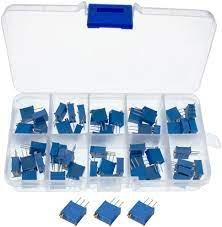10 various Potentiometer variable resistors (50PCS)

# 10 various Potentiometer variable resistors (50PCS)

\$14.00

In stock\$14.00

In stock

SKU: #SB-A-00128 Categories: , Tags: ,

Share:

10 various Potentiometer variable resistorsCode / Values

```102 = 1K ohm
103 = 10K ohm
104 = 100K ohm
105 = 1M ohm
202 = 2K ohm
203 = 20K ohm
204 = 200K ohm
501 = 500R ohm
502 = 5K ohm
503 = 50K ohm```
Each 5PCS and in a box
Packing list:
50 pcs : 5pcs x 10 different values as above